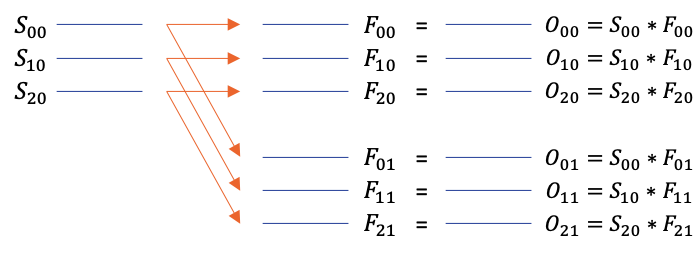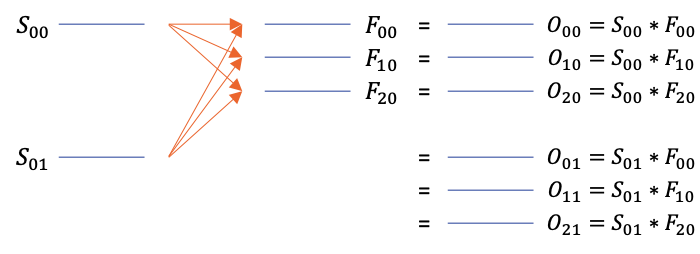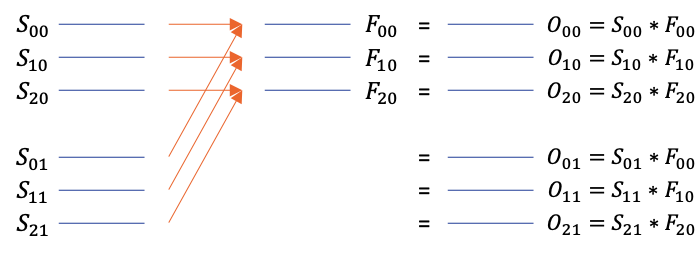A high-performance general-purpose compute library

Convolution Integral for two dimensional data. More...

## Functions

AFAPI array convolve2 (const array &signal, const array &filter, const convMode mode=AF_CONV_DEFAULT, const convDomain domain=AF_CONV_AUTO)
C++ Interface for convolution on two dimensional signals.

AFAPI array convolve2NN (const array &signal, const array &filter, const dim4 stride, const dim4 padding, const dim4 dilation)
C++ Interface for 2D convolution.

AFAPI array fftConvolve2 (const array &signal, const array &filter, const convMode mode=AF_CONV_DEFAULT)
C++ Interface for convolution on 2D signals using FFT.

AFAPI af_err af_convolve2 (af_array *out, const af_array signal, const af_array filter, const af_conv_mode mode, af_conv_domain domain)
C Interface for convolution on two dimensional signals.

AFAPI af_err af_convolve2_nn (af_array *out, const af_array signal, const af_array filter, const unsigned stride_dims, const dim_t *strides, const unsigned padding_dims, const dim_t *paddings, const unsigned dilation_dims, const dim_t *dilations)
C Interface for 2D convolution.

AFAPI af_err af_fft_convolve2 (af_array *out, const af_array signal, const af_array filter, const af_conv_mode mode)
C Interface for convolution on 2D signals using FFT.

## Detailed Description

Convolution Integral for two dimensional data.

A convolution is a common operation between a source array, a, and a filter (or kernel) array b. The answer to the convolution is the same as computing the coefficients in polynomial multiplication, if a and b are the coefficients.

Another way to think about it is that the filter kernel is centered on each pixel in a, and the output for that pixel or data point is the sum of the products.

Depending on the size of the signal and the filter, any one of the following batch mode convolutions take place.

• No Batch - Single filter applied to single input.
• Filter is Batched - Many filters applied on same input
• Signal is Batched - Single filter applied to a set of inputs.
• Identical Batches - A set of filters applied onto to a set of inputs in one-to-one correspondence.
• Non overlapping Batches - All batched filters are applied to all batched signals. The batch axis of Signal and Filter should not be the same.
Note
All non-overlapping(interleaved) convolutions default to frequency domain AF_CONV_FREQ irrespective of the provided convolution mode argument.

A detailed explanation of each batch mode for 2D convolutions is provided below. Given below are definitions of variables and constants that are used to facilitate easy illustration of the operations.

• $$[M\quad N]$$, $$[A\quad B]$$ are signal, filter sizes along $$0^{th}$$ & $$1^{st}$$ axes respectively.
• $$P$$ and $$Q$$ are two constants, integers greater than one.
• $$p$$ is an integer variable with range $$\ 0 \leq p < P$$.
• $$q$$ is an integer variable with range $$\ 0 \leq q < Q$$.
• O, S and F are notations for Output, Signal and Filter respectively.

We have also used images to showcase some examples which follow the below notation.

• Each blue line is a two dimensional matrix.
• Each orange line indicates a full 2d convolution operation.
• Suffix of each letter indicates indices along $$3^{rd}$$ and $$4^{th}$$ axes in the order of appearance from left to right in the suffix.
• O, S and F are notations for Output, Signal and Filter respectively.

### No Batch

Given below is an example of no batch mode.Single 2d convolution with 2d filter

For input size $$[M \ N \ 1 \ 1]$$ and filter size $$[A \ B \ 1 \ 1]$$, the following set-builder notation gives a formal definition of all convolutions performed in this mode.

$$\underset{[M \ N \ 1 \ 1]}{O}$$ = $$\underset{[M \ N \ 1 \ 1]}{S} \ast \underset{[A \ B \ 1 \ 1]}{F}$$

### Batched Filter

Given below is an example of filter batch mode.Single signal convolved with many filters independently

For input size $$[M \ N \ 1 \ 1]$$ and filter size $$[A \ B \ P \ 1]$$, the following set-builder notation gives a formal definition of all convolutions performed in this mode.

$$\underset{[M \ N \ P \ 1]}{O}$$ = $$\left\{ \underset{[M \ N \ 1 \ 1]}{S} \ast \underset{[A \ B \ p \ 1]}{f} \ \Bigg\vert \ \forall \underset{[A \ B \ p \ 1]}{f} \in \underset{[A \ B \ P \ 1]}{F} \right\}$$

### Batched Signal

Given below is an example of signal batch mode.Single filter convolved with many signals independently

For input size $$[M \ N \ P \ 1]$$ and filter size $$[A \ B \ 1 \ 1]$$, the following set-builder notation gives a formal definition of all convolutions performed in this mode.

$$\underset{[M \ N \ P \ 1]}{O}$$ = $$\left\{ \underset{[M \ N \ p \ 1]}{s} \ast \underset{[A \ B \ 1 \ 1]}{F} \ \Bigg\vert \ \forall \underset{[M \ N \ p \ 1]}{s} \in \underset{[M \ N \ P \ 1]}{S} \right\}$$

### Identical Batch Sizes

Given below is an example of identical batch mode.Many signals convolved with many filters in one-on-one manner

For input size $$[M \ N \ P \ Q]$$ and filter size $$[A \ B \ P \ Q]$$, the following set-builder notation gives a formal definition of all convolutions performed in this mode.

$$\underset{[M \ N \ P \ Q]}{O}$$ = $$\left\{ \underset{[M \ N \ p \ q]}{s} \ast \underset{[A \ B \ p \ q]}{f} \ \Bigg\vert \ \forall \underset{[M \ N \ p \ q]}{s} \in \underset{[M \ N \ P \ Q]}{S} \land \forall \underset{[M \ N \ p \ q]}{f} \in \underset{[M \ N \ P \ Q]}{F} \right\}$$

### Non-overlapping Batches

Four different kinds of signal and filter size combinations are handled in this batch mode. Each one of them are explained in respective sections below.

#### Combination 1

For input size $$[M \ N \ P \ 1]$$ and filter size $$[A \ B \ 1 \ Q]$$, the following set-builder notation gives a formal definition of all convolutions performed in this mode.

$$\underset{[M \ N \ P \ Q]}{O}$$ = $$\left\{ \left\{ \underset{[M \ N \ p \ 1]}{s} \ast \underset{[A \ B \ 1 \ q]}{f} \ \Bigg\vert \ \forall \underset{[M \ N \ p \ 1]}{s} \in \underset{[M \ N \ P \ 1]}{S} \right\} \ \Bigg\vert \ \forall \underset{[A \ B \ 1 \ q]}{f} \in \underset{[A \ B \ 1 \ Q]}{F} \right\}$$

Given below is an example of this batch mode.#### Combination 2

For input size $$[M \ N \ P \ 1]$$ and filter size $$[A \ B \ P \ Q]$$, the following set-builder notation gives a formal definition of all convolutions performed in this mode.

$$\underset{[M \ N \ P \ Q]}{O}$$ = $$\left\{ \left\{ \underset{[M \ N \ p \ 1]}{s} \ast \underset{[A \ B \ p \ q]}{f} \ \Bigg\vert \ \forall \underset{[A \ B \ p \ q]}{f} \in \underset{[A \ B \ P \ Q]}{F} \right\} \ \Bigg\vert \ \forall \underset{[M \ N \ p \ 1]}{s} \in \underset{[M \ N \ P \ 1]}{S} \right\}$$

Given below is an example of this batch mode.#### Combination 3

For input size $$[M \ N \ 1 \ P]$$ and filter size $$[A \ B \ Q \ 1]$$, the following set-builder notation gives a formal definition of all convolutions performed in this mode.

$$\underset{[M \ N \ Q \ P]}{O}$$ = $$\left\{ \left\{ \underset{[M \ N \ 1 \ p]}{s} \ast \underset{[A \ B \ q \ 1]}{f} \ \Bigg\vert \ \forall \underset{[M \ N \ 1 \ p]}{s} \in \underset{[M \ N \ 1 \ P]}{S} \right\} \ \Bigg\vert \ \forall \underset{[A \ B \ q \ 1]}{f} \in \underset{[A \ B \ Q \ 1]}{F} \right\}$$

Given below is an example of this batch mode.#### Combination 4

For input size $$[M \ N \ P \ Q]$$ and filter size $$[A \ B \ P \ 1]$$, the following set-builder notation gives a formal definition of all convolutions performed in this mode.

$$\underset{[M \ N \ P \ Q]}{O}$$ = $$\left\{ \left\{ \underset{[M \ N \ p \ q]}{s} \ast \underset{[A \ B \ p \ 1]}{f} \ \Bigg\vert \ \forall \underset{[M \ N \ p \ q]}{s} \in \underset{[M \ N \ P \ Q]}{S} \right\} \ \Bigg\vert \ \forall \underset{[A \ B \ p \ 1]}{f} \in \underset{[A \ B \ P \ 1]}{F} \right\}$$

Given below is an example of this batch mode.The batching behavior of convolve2NN functions(af_convolve2_nn() and af::convolve2NN() ) is different from convolve2. The new functions can perform 2D convolution on 3D signals and filters in a way that is more aligned with convolutional neural networks.

Signal Size Filter Size Output Size Batch Mode Description
$$[M \ N \ 1 \ 1]$$ $$[M \ N \ 1 \ 1]$$ $$[M \ N \ 1 \ 1]$$ No Batch Output will be a single convolved array
$$[M \ N \ 1 \ 1]$$ $$[M \ N \ P \ 1]$$ $$[M \ N \ P \ 1]$$ Invalid Size along second axis should be same
$$[M \ N \ P \ 1]$$ $$[M \ N \ 1 \ 1]$$ $$[M \ N \ P \ 1]$$ Invalid Size along second axis should be same
$$[M \ N \ P \ 1]$$ $$[M \ N \ P \ 1]$$ $$[M \ N \ 1 \ 1]$$ No Batch 3D Signal and 3D filter convoled to 2D result
$$[M \ N \ P \ Qs]$$ $$[M \ N \ P \ Qf]$$ $$[M \ N \ Qf \ Qs]$$ Batch Qs * Qf Qs signals and Qf filters to create Qs * Qf results
Note
For the above tabular illustrations, we will assume af_conv_mode is AF_CONV_DEFAULT.

## ◆ af_convolve2()

 AFAPI af_err af_convolve2 ( af_array * out, const af_array signal, const af_array filter, const af_conv_mode mode, af_conv_domain domain )

C Interface for convolution on two dimensional signals.

Parameters
 [out] out is convolved array [in] signal is the input signal [in] filter is the signal that shall be flipped for the convolution operation [in] mode indicates if the convolution should be expanded or not(where output size equals input) [in] domain specifies if the convolution should be performed in frequency os spatial domain
Returns
AF_SUCCESS if the convolution is successful, otherwise an appropriate error code is returned.
Note
The default parameter of domain, AF_CONV_AUTO, heuristically switches between frequency and spatial domain.

## ◆ af_convolve2_nn()

 AFAPI af_err af_convolve2_nn ( af_array * out, const af_array signal, const af_array filter, const unsigned stride_dims, const dim_t * strides, const unsigned padding_dims, const dim_t * paddings, const unsigned dilation_dims, const dim_t * dilations )

C Interface for 2D convolution.

This version of convolution is consistent with the machine learning formulation that will spatially convolve a filter on 2-dimensions against a signal. Multiple signals and filters can be batched against each other. Furthermore, the signals and filters can be multi-dimensional however their dimensions must match.

Example: Signals with dimensions: d0 x d1 x d2 x Ns Filters with dimensions: d0 x d1 x d2 x Nf

Resulting Convolution: d0 x d1 x Nf x Ns

Parameters
 [out] out is convolved array [in] signal is the input signal [in] filter is the filter that will be used for the convolution operation [in] stride_dims specifies the number of stride dimension parameters [in] strides array of values specifying the amounts the filter strides along each dimension [in] padding_dims specifies the number of padding dimension parameters [in] paddings array of values specifying the amounts to pad along each dimension [in] dilation_dims specifies the number of dilation dimension parameters [in] dilations array of values specifying the amounts to dilate the filter before convolving along each dimension
Returns
AF_SUCCESS if the convolution is successful, otherwise an appropriate error code is returned.

## ◆ af_fft_convolve2()

 AFAPI af_err af_fft_convolve2 ( af_array * out, const af_array signal, const af_array filter, const af_conv_mode mode )

C Interface for convolution on 2D signals using FFT.

Parameters
 [out] out is convolved array [in] signal is the input signal [in] filter is the signal that shall be used for the convolution operation [in] mode indicates if the convolution should be expanded or not(where output size equals input)
Returns
AF_SUCCESS if the convolution is successful, otherwise an appropriate error code is returned.

## ◆ convolve2()

 AFAPI array convolve2 ( const array & signal, const array & filter, const convMode mode = AF_CONV_DEFAULT, const convDomain domain = AF_CONV_AUTO )

C++ Interface for convolution on two dimensional signals.

// vector<dim4> numDims;
// vector<vector<float> > in;
array signal(numDims, &(in.front()));
// signal dims = [15 17 1 1]
array filter(numDims, &(in.front()));
// filter dims = [5 5 2 1]
array output = convolve2(signal, filter, AF_CONV_DEFAULT);
// output dims = [15 17 1 1] - same as input since expand(3rd argument is
// false) however, notice that the 3rd dimension of filter is > 1. So, one
// to many batch mode will be activated automatically where the 2d input
// signal is convolved with each 2d filter and the result will written
// corresponding slice in the output 3d array
A multi dimensional data container.
Definition: array.h:37
@ AF_CONV_DEFAULT
Output of the convolution is the same size as input.
Definition: defines.h:305
AFAPI array convolve2(const array &signal, const array &filter, const convMode mode=AF_CONV_DEFAULT, const convDomain domain=AF_CONV_AUTO)
C++ Interface for convolution on two dimensional signals.
AFAPI array filter(const array &image, const array &kernel)
Image Filtering.
Parameters
 [in] signal is the input signal [in] filter is the signal that shall be flipped for the convolution operation [in] mode indicates if the convolution should be expanded or not(where output size equals input) [in] domain specifies if the convolution should be performed in frequency os spatial domain
Returns
the convolved array
Note
The default parameter of domain, AF_CONV_AUTO, heuristically switches between frequency and spatial domain.

## ◆ convolve2NN()

 AFAPI array convolve2NN ( const array & signal, const array & filter, const dim4 stride, const dim4 padding, const dim4 dilation )

C++ Interface for 2D convolution.

This version of convolution is consistent with the machine learning formulation that will spatially convolve a filter on 2-dimensions against a signal. Multiple signals and filters can be batched against each other. Furthermore, the signals and filters can be multi-dimensional however their dimensions must match.

Example: Signals with dimensions: d0 x d1 x d2 x Ns Filters with dimensions: d0 x d1 x d2 x Nf

Resulting Convolution: d0 x d1 x Nf x Ns

Parameters
 [in] signal is the input signal [in] filter is the filter that will be used for the convolution operation [in] stride specifies the filter strides along each dimension [in] padding specifies the padding along each dimension [in] dilation specifies the amount to dilate the filter before convolution
Returns
the convolved array
Note
Make sure you pass in both dim0, and dim1 in your dim4 arguments. The third and fourth dimensions are currently ignored.

## ◆ fftConvolve2()

 AFAPI array fftConvolve2 ( const array & signal, const array & filter, const convMode mode = AF_CONV_DEFAULT )

C++ Interface for convolution on 2D signals using FFT.

Parameters
 [in] signal is the input signal [in] filter is the signal that shall be used for the convolution operation [in] mode indicates if the convolution should be expanded or not(where output size equals input)
Returns
the convolved array